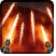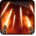# FlurryConditions

Used by

## Related effects, buffs and debuffs

Please click on an effect below to view its details.

• [0s] [not displayed]Effect #1

 Slot: Debuff Duration: 0s Tick rate: does not tick # occurrences: 0 Tags: tag.​abl.​utility.​is_basic_attack
• On Apply

Only when the following conditions are met:

• If CASTER is positioned not more than 4m to melee distance of TARGET

Perform the following actions:

• Add effect #2 to TARGET from TARGET
• On Apply

Only when the following conditions are met:

• <
• If PRIMARY TARGET has an effect with the tag tag.​abl.​exp.​seasons.​02.​ep_01.​walker.​is_in_walker
AND
• If CASTER is positioned 4.001m to 60m to melee distance of TARGET
AND
• Unknown (84)
- Negated = (bool) false
- Block Identifier = (int) 1
>

Perform the following actions:

• Add effect #4 to TARGET from TARGET
• On Apply

Only when the following conditions are met:

• <
• If CASTER is positioned 4.001m to 60m to melee distance of TARGET
AND
• Unknown (84)
- Negated = (bool) false
- Block Identifier = (int) 1
>

Perform the following actions:

• Add effect #3 to TARGET from TARGET
• [0s] [not displayed]Effect #2

 Slot: Debuff Duration: 0s Tick rate: does not tick # occurrences: 0 Tags: tag.​abl.​utility.​is_basic_attack Conditions: Can only by called by other effects
• On Apply

Only when the following conditions are met:

• <
• If PRIMARY TARGET has an effect with the tag tag.​abl.​exp.​seasons.​02.​ep_01.​walker.​is_in_walker
AND
• Unknown (84)
- Negated = (bool) false
- Block Identifier = (int) 1
>

Perform the following actions:

• Play appearance epp . flurry . droid . atst . melee_flurry
• Spell Damage
- Unknown (609) = (bool) false
- Damage Type = (int) 1
- Slot = (int) 1
- Spell Type = (int) 3
- Level Cap = (int) 0
- Amount Max = (float) 0
- Amount Min = (float) 0
- Amount Percent = (float) 0
- Threat Percent = (float) 0
- Standard Health Percent Max = (float) 0.4
- Standard Health Percent Min = (float) 0.4
- Amount Modifier Percent = (float) 0
- Coefficient = (float) 0
- Health Steal Percentage = (float) 0
• On Apply

Only when the following conditions are met:

• Unknown (84)
- Negated = (bool) false
- Block Identifier = (int) 1

Perform the following actions:

• Play appearance epp . flurry . droid . atst . melee_flurry
• Spell Damage
- Unknown (609) = (bool) false
- Damage Type = (int) 1
- Slot = (int) 1
- Spell Type = (int) 2
- Level Cap = (int) 0
- Amount Max = (float) 0
- Amount Min = (float) 0
- Amount Percent = (float) 0
- Threat Percent = (float) 0
- Standard Health Percent Max = (float) 0.025
- Standard Health Percent Min = (float) 0.025
- Amount Modifier Percent = (float) 0
- Coefficient = (float) 0
- Health Steal Percentage = (float) 0
• [hidden] [0.5s]Effect #3

 Slot: Debuff Duration: 0.5s Tick rate: 0.1s # occurrences: 0 Tags: tag.​abl.​utility.​is_basic_attack Conditions: Can only by called by other effects
• On Apply

Perform the following actions:

• Play appearance epp . world_design . polymorph_abilities . atst_skeleton . flurry_ranged
• When effect ticks the 1th time

Perform the following actions:

• Spell Damage
- Unknown (609) = (bool) false
- Damage Type = (int) 1
- Slot = (int) 1
- Spell Type = (int) 2
- Level Cap = (int) 0
- Amount Max = (float) 0
- Amount Min = (float) 0
- Amount Percent = (float) 0
- Threat Percent = (float) 0
- Standard Health Percent Max = (float) 0.05
- Standard Health Percent Min = (float) 0.05
- Amount Modifier Percent = (float) 0
- Coefficient = (float) 0
- Health Steal Percentage = (float) 0
• When effect ticks the 2th time

Perform the following actions:

• Spell Damage
- Unknown (609) = (bool) false
- Damage Type = (int) 1
- Slot = (int) 1
- Spell Type = (int) 2
- Level Cap = (int) 0
- Amount Max = (float) 0
- Amount Min = (float) 0
- Amount Percent = (float) 0
- Threat Percent = (float) 0
- Standard Health Percent Max = (float) 0.05
- Standard Health Percent Min = (float) 0.05
- Amount Modifier Percent = (float) 0
- Coefficient = (float) 0
- Health Steal Percentage = (float) 0
• When effect ticks the 4th time

Perform the following actions:

• Spell Damage
- Unknown (609) = (bool) false
- Damage Type = (int) 1
- Slot = (int) 1
- Spell Type = (int) 2
- Level Cap = (int) 0
- Amount Max = (float) 0
- Amount Min = (float) 0
- Amount Percent = (float) 0
- Threat Percent = (float) 0
- Standard Health Percent Max = (float) 0.05
- Standard Health Percent Min = (float) 0.05
- Amount Modifier Percent = (float) 0
- Coefficient = (float) 0
- Health Steal Percentage = (float) 0
• When effect ticks the 5th time

Perform the following actions:

• Spell Damage
- Unknown (609) = (bool) false
- Damage Type = (int) 1
- Slot = (int) 1
- Spell Type = (int) 2
- Level Cap = (int) 0
- Amount Max = (float) 0
- Amount Min = (float) 0
- Amount Percent = (float) 0
- Threat Percent = (float) 0
- Standard Health Percent Max = (float) 0.05
- Standard Health Percent Min = (float) 0.05
- Amount Modifier Percent = (float) 0
- Coefficient = (float) 0
- Health Steal Percentage = (float) 0
• [hidden] [0.5s]Effect #4

 Slot: Debuff Duration: 0.5s Tick rate: 0.1s # occurrences: 0 Tags: tag.​abl.​utility.​is_basic_attack Conditions: Can only by called by other effects
• On Apply

Perform the following actions:

• Play appearance epp . world_design . polymorph_abilities . atst_skeleton . flurry_ranged
• When effect ticks the 1th time

Perform the following actions:

• Spell Damage
- Unknown (609) = (bool) false
- Damage Type = (int) 1
- Slot = (int) 1
- Spell Type = (int) 2
- Level Cap = (int) 0
- Amount Max = (float) 0
- Amount Min = (float) 0
- Amount Percent = (float) 0
- Threat Percent = (float) 0
- Standard Health Percent Max = (float) 0.2
- Standard Health Percent Min = (float) 0.2
- Amount Modifier Percent = (float) 0
- Coefficient = (float) 0
- Health Steal Percentage = (float) 0
• When effect ticks the 2th time

Perform the following actions:

• Spell Damage
- Unknown (609) = (bool) false
- Damage Type = (int) 1
- Slot = (int) 1
- Spell Type = (int) 2
- Level Cap = (int) 0
- Amount Max = (float) 0
- Amount Min = (float) 0
- Amount Percent = (float) 0
- Threat Percent = (float) 0
- Standard Health Percent Max = (float) 0.2
- Standard Health Percent Min = (float) 0.2
- Amount Modifier Percent = (float) 0
- Coefficient = (float) 0
- Health Steal Percentage = (float) 0
• When effect ticks the 4th time

Perform the following actions:

• Spell Damage
- Unknown (609) = (bool) false
- Damage Type = (int) 1
- Slot = (int) 1
- Spell Type = (int) 2
- Level Cap = (int) 0
- Amount Max = (float) 0
- Amount Min = (float) 0
- Amount Percent = (float) 0
- Threat Percent = (float) 0
- Standard Health Percent Max = (float) 0.2
- Standard Health Percent Min = (float) 0.2
- Amount Modifier Percent = (float) 0
- Coefficient = (float) 0
- Health Steal Percentage = (float) 0
• When effect ticks the 5th time

Perform the following actions:

• Spell Damage
- Unknown (609) = (bool) false
- Damage Type = (int) 1
- Slot = (int) 1
- Spell Type = (int) 2
- Level Cap = (int) 0
- Amount Max = (float) 0
- Amount Min = (float) 0
- Amount Percent = (float) 0
- Threat Percent = (float) 0
- Standard Health Percent Max = (float) 0.2
- Standard Health Percent Min = (float) 0.2
- Amount Modifier Percent = (float) 0
- Coefficient = (float) 0
- Health Steal Percentage = (float) 0